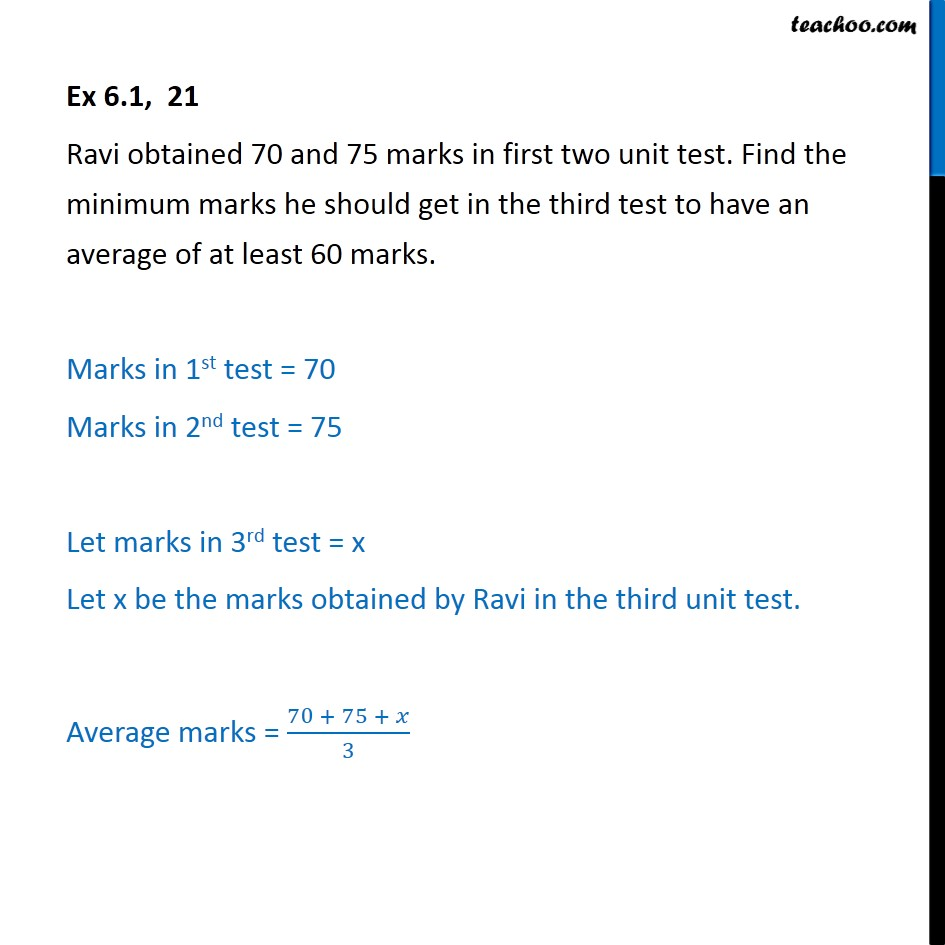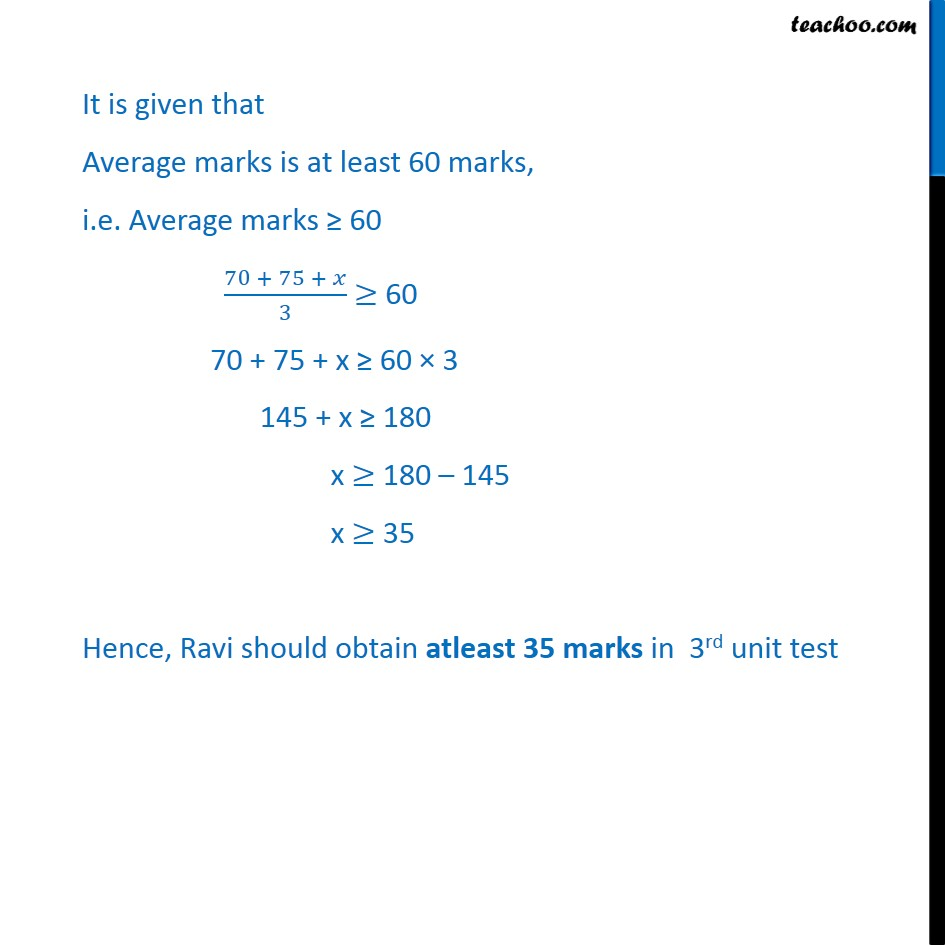Ex 5.1

Chapter 5 Class 11 Linear Inequalities
Serial order wiseLearn in your speed, with individual attention - Teachoo Maths 1-on-1 Class

### Transcript

Ex 5.1, 21 Ravi obtained 70 and 75 marks in first two unit test. Find the minimum marks he should get in the third test to have an average of at least 60 marks. Marks in 1st test = 70 Marks in 2nd test = 75 Let marks in 3rd test = x Let x be the marks obtained by Ravi in the third unit test. Average marks = ﷐70 + 75 + 𝑥﷮3﷯ It is given that Average marks is at least 60 marks, i.e. Average marks ≥ 60 ﷐70 + 75 + 𝑥﷮3﷯ ≥ 60 70 + 75 + x ≥ 60 × 3 145 + x ≥ 180 x ≥ 180 – 145 x ≥ 35 Hence, Ravi should obtain atleast 35 marks in 3rd unit test